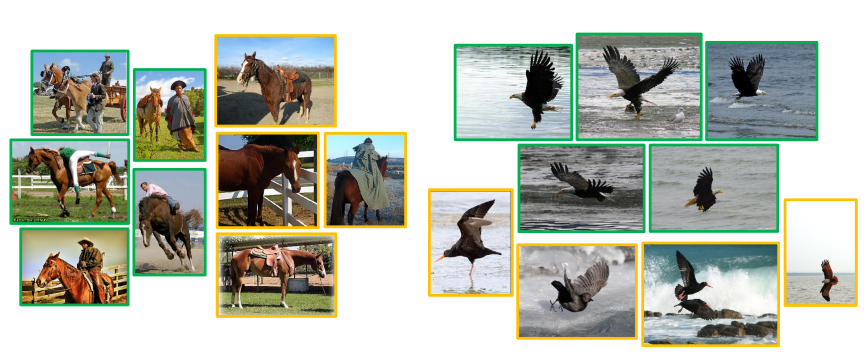code: https://github.com/salesforce/PCL

# Contribution

1、在自监督对比学习的特征空间引入聚类，聚类希望语义相似的负样本实例约束在同一个原型群组中，不会因为对比损失而拉大差距；

2、提出原型的概念，一个原型是指相似语义结构的特征表示聚在一个群组（图中的圆）中的embedding；即使是负样本，语义结构相似的应该属于同一群组（同一个圆内）。

3、为了估计每个原型群组中的特征分布，文章把泛化InfoNCE loss为ProtoNCE loss；

4、将整个数据集的图像分配给不同粒度的多个原型群组。# 做法

## EM算法

EM算法主要解决在极大似然估计中，输入数据的概率分布未知的问题，通过引入隐变量$𝒛$的分布，方便对参数$\theta$进行极大似然估计。

• E Step：隐变量的条件概率分布的期望的求解——根据当前的模型参数$\theta^{(i)}$和观测数据$X$求得隐变量的条件概率分布期望$Q(z^{(i)})$；
• M Step：参数的求解——根据$Q(z^{(i)})$求参数$\theta^{(i)}$的似然函数，通过取对数求导，令导数等于0得到模型参数$\theta^{(i+1)}$。
重复上述操作。直到$\theta$收敛。

$$L_{ProtoNCE}=L_{infoNCE} + L_{Proto}$$

## 网络参数具体求解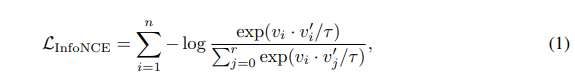$n$张图像的极大似然估计为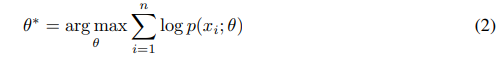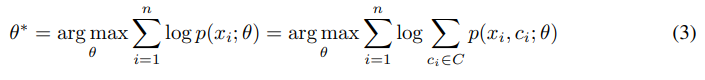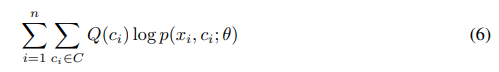### M step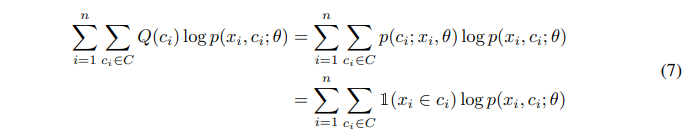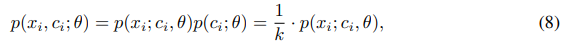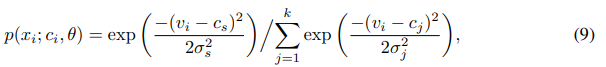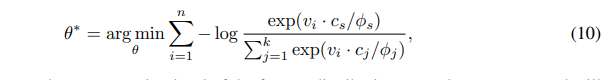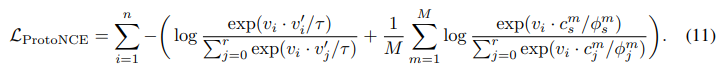### 浓度系数估计momentum编码器的输出特征为$\{v_z^{\prime}\}_{z=1}^Z$。

# 实验结果

## TSNE图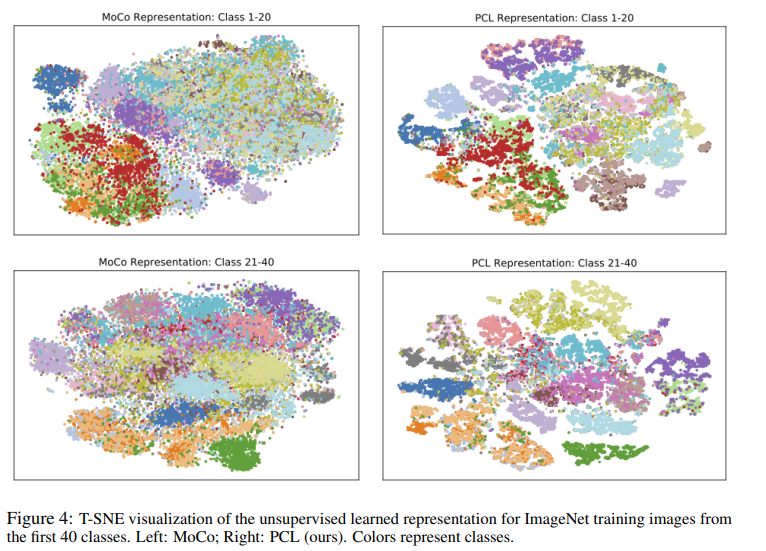## 粗细粒度分类展示